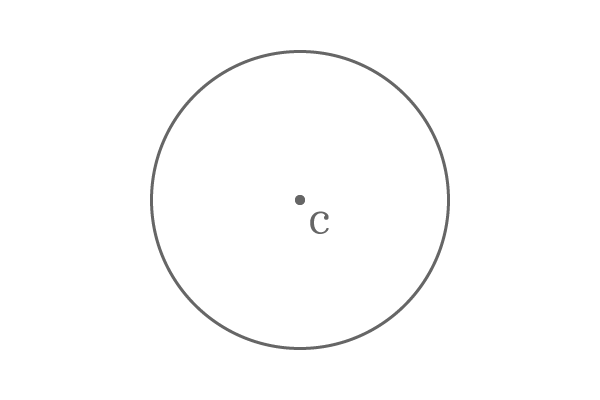# Centre of a circle

## Definition

An inside point that maintains equal distance from all the points on the circumference of a circle is called the centre of a circle.There are numerous points inside every circle but a particular point is exactly middle to the circle.

The middle point of the circle maintains same distance from every point on the circumference of the circle. So, the point is known as centre of the circle.

The centre of a circle is a point geometrically. So, it is also represented same as the notation of the point.

### ExamplePoint $C$ is an inside point of the circle and it maintains equal distance from every point on the circumference of the same circle.

Therefore, the point $C$ is called as centre of the circle.

Latest Math Topics
Latest Math Problems
Email subscription
Math Doubts is a best place to learn mathematics and from basics to advanced scientific level for students, teachers and researchers. Know more